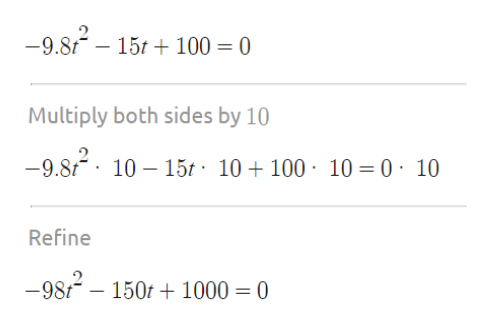# Quadratic equations are used for many applications. The formula to solve quadratic equations can be used to solve all quadratic equations, even those that cannot be factored. A common application of quadratic equations is the height of a thrown object over time, which takes the earth's gravity and the velocity of the throw into account. For example, if someone throws a ball down from a height of 100 meters, the ball's distance from the ground can be modeled by the equation: d = − 9.8 t 2 − 15 t + 100 where t is the time in seconds and d is the distance in meters.At what time (t) will the ball hit the ground? (Hint: what does this mean for d=distance?)You will get two answers. Do both make sense? (Explain in detail Why or Why not)...

Question
22 views

Quadratic equations are used for many applications. The formula to solve quadratic equations can be used to solve all quadratic equations, even those that cannot be factored. A common application of quadratic equations is the height of a thrown object over time, which takes the earth's gravity and the velocity of the throw into account. For example, if someone throws a ball down from a height of 100 meters, the ball's distance from the ground can be modeled by the equation: d = − 9.8 t 2 − 15 t + 100 where t is the time in seconds and d is the distance in meters.

1. At what time (t) will the ball hit the ground? (Hint: what does this mean for d=distance?)
2. You will get two answers. Do both make sense? (Explain in detail Why or Why not)...
check_circle

Step 1

When the ball hits the ground then d=0

From there we have to solve for t.

Before that we simplify the equation to remove the decimal.help_outlineImage Transcriptionclose-9.8 15t100 0 Multiply both sides by 10 -9.810 15t - 10+100 10 = 0 10 Refine -98/150r1000 = 0 fullscreen
Step 2

Then we solve for t using quadratic formula.

t= -4.05 and t=2.52

Time can not be negative ...help_outlineImage Transcriptionclose-(-160) ± V (-150)- - 4(-98)1000 2(-98) For a= -98, b= -150, c = 1000: 12= 5(15 145 -(-150) 150)- 4(-98)1000 2(-98) t= 98 -(-150) V150)-4(-98)1000 2(-98) 51415) 98 t= The solutions to the quadratic equation are: 5(15+ 4145 98 5414515) t= t= 98 t- -4.05 t-2.52 fullscreen

### Want to see the full answer?

See Solution

#### Want to see this answer and more?

Solutions are written by subject experts who are available 24/7. Questions are typically answered within 1 hour.*

See Solution
*Response times may vary by subject and question.
Tagged in

### Other